Importance: High ✭✭✭
 Author(s): Aharoni, Ron Berger, Eli
 Subject: Combinatorics » Matroid Theory
 Keywords: independent set matroid partition
 Posted by: mdevos on: June 11th, 2007
Conjecture   If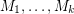are matroids on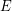and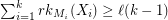for every partitionof, then there existswith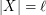which is independent in every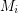.

Let us begin by stating two classic results. For a graph (or hypergraph) we letdenote the size of the smallest (vertex) cover and we letdenote the size of the largest matching.

Theorem  (König)for every bipartite graph.
Theorem  (Matroid Intersection)   Ifare matroids onandfor every partition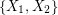of, then there existswithwhich is independent in both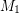and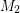.

The matroid intersection theorem is exactly the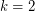case of the above conjecture, but it may also be viewed as a generalization of König's theorem. To see this, letbe a bipartite graph with edge setand bipartition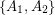and forletbe the (uniform) matroid onwhere a subset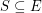is independent if no two edges inshare an endpoint in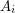. Thenis the number of vertices inwhich are incident with an edge in, so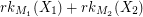has minimum value, and a set of edges is independent in bothandif and only if it is a matching, so the size of the largest such set is.

A famous conjecture of Ryser suggests a generalization of König's theorem to hypergraphs. It claims that every-partite-uniform hypergraph satisfies. The above conjecture is the common generalization of this conjecture of Ryser and the matroid intersection theorem. Aharoni [A] proved the 3-partite 3-uniform case of Ryser's conjecture, and this was extended by Aharoni-Berger [AB] to thecase of the above conjecture. The conjecture remains open for.

## Bibliography

[A] R. Aharoni, Ryser's conjecture for tripartite 3-graphs, Combinatorica 21 (2001), 1-4. MathSciNet

*[AB] R. Aharoni, E. Berger, The intersection of a matroid with a simplicial complex. Trans. Amer. Math. Soc. 358 (2006), no. 11 MathSciNet

* indicates original appearance(s) of problem.